# Improving NI RF System Accuracy with Methodical Calibration Techniques

## Visão geral

Today’s RF electronic devices pose many challenges for businesses tasked with ensuring that quality products are fabricated according to specifications. Demand for RF products such as cellular phones, wireless local area network (WLAN) adapters, and portable GPS receivers continues to grow, correspondingly driving demand for low-level RF components such as RF power amplifiers and mixers.

In an environment where the testing coverage needed for an individual RF device during characterization, verification, validation, and manufacturing test has increased, both test speed and measurement accuracy are being pushed to achieve better results across larger numbers of test stations over longer periods of time.

Test speed and measurement accuracy are normally conflicting goals. This is certainly the case when making RF power measurements. Better power measurement accuracy and repeatability typically require longer test times due to differing instrumentation (for example, use of a power meter versus a signal analyzer) or measurement settings (for example, averaging), and test times are often reduced at the expense of power measurement accuracy to increase throughput.

RF system calibration offers a solution to these dueling requirements of speed and accuracy. The following discussion looks at a commonly used simple method for calibrating an RF signal generator and signal analyzer system and this method’s associated drawbacks. Sources of measurement error are identified and quantified, with techniques to reduce the larger contributors discussed. Finally, a methodical procedure for using a power meter as a reference for calibrating an RF signal analyzer and signal generator measurement system is detailed, so you can achieve RF power measurement accuracy performance similar to a power meter while still retaining the faster measurement speed and greater dynamic range of a signal analyzer.

NI RF instruments feature strong repeatability and relative accuracy performance. However, all RF vector signal analyzers (VSAs) and RF vector signal generators (VSGs) exhibit inferior absolute amplitude accuracy compared to an RF power meter. This document explains the pitfalls inherent in an uncalibrated VSG/VSA system, the benefits of using external attenuator padding, and a calibration procedure that you can use to improve the amplitude accuracy of RF measurements by calibrating a VSA/VSG RF measurement system using a power meter as a reference. Variables that affect amplitude accuracy are discussed, and an error model for combining measurement uncertainties to calculate overall measurement uncertainty is covered. Also included is an automated NI TestStand sequence that you can use to calibrate an NI PXIe-5663/PXIe-5673 6.6 GHz RF measurement system using the NI USB-5680 RF power meter as a measurement reference. Required hardware to use this sequence includes the following: 1. NI PXIe-5663 RF vector signal analyzer 2. NI PXIe-5673 RF vector signal generator 3. NI USB-5680 4. F SMA-F SMA barrel adapter 5. Two SMA-SMA cables 6. Two coaxial SMA attenuators (not required, but HIGHLY recommended)

## Simple Approach With Signal Analyzer

### Simple Approach With Signal Analyzer

Consider the simple test setup in Figure 1. In this setup, the user directly connects the RF signal generator to the unit under test’s (UUT’s) RF port. An expensive, low-loss coaxial cable is often used, but there is still a loss associated with the cable that is a function of frequency, Lc(f). To present a consistent signal level over frequency to the unit under test’s RF port, Prx, the output signal level of the RF signal generator, Psg, is programmed as the following: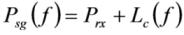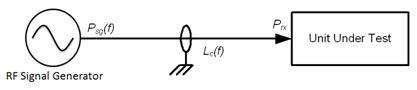Figure 1. Initial Setup

Although an ideal situation with minimal RF connections, this setup has many pitfalls. A considerable source of error comes from the RF signal generator's output level uncertainty, especially at low RF power levels.

Figure 2 shows the level of uncertainty for the NI PXIe-5673 signal generator.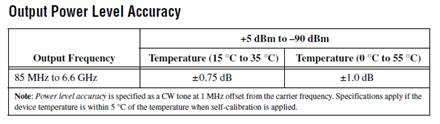Figure 2. NI PXIe-5673 Power Accuracy (numbers are absolute maximums)

The generator's ability to meet the stated performance has some limitations. Many generators’ data sheets specify ambient temperature ranges that the generator must operate within to achieve stated accuracy performance. These ranges are typically a small window of temperature deviations from room temperature (25 ˚C). Given the typical manufacturing environment with poor HVAC systems and seasonal temperature changes, it is plausible for the temperature to vary +10 °C.

Another inherent problem with the setup in Figure 1 is impedance mismatch error caused by differences in impedance between the generator and the unit under test. Mismatch errors are always an important source of error in RF power measurements, and they typically are the largest contributor of measurement uncertainty. The following discussion of mismatch errors in Figure 1 makes these assumptions:

• The return loss of cables and adapters is insignificant (in other words, only the VSWRs/impedances of the RF signal generator and UUT are considered).
• The mismatch error caused by impedance mismatch between the RF signal generator and the power meter is ignored since power sensors typically have return loss <  -25 dB. This overall contribution is very small relative to the mismatch error between the RF generator and the UUT.
• The VSWR specified for the Rohde & Schwarz SMIQ for f >2.0 GHz is less than 2.0 while the NI PXIe-5673 VSWR is specified as 1.9 for output levels <-10 dBm. For this discussion, a VSWR of 1.9 is used.

UUTs often have poor VSWR. For this example, assume a UUT VSWR of 2.5. In calculating the mismatch error, refer to Figure 3. Using the prior assumptions, the mismatch uncertainties between reference planes A0 (VSWR = 1.9) and B0 (VSWR = 2.5) are the following: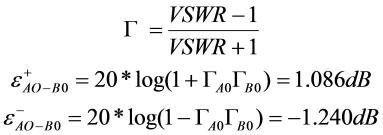Therefore, the uncertainty due to poor impedance matching is approximately -1.25 to +1.10 dB.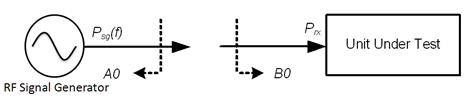Figure 3. Reference Planes

Many users resort to calibrating their systems to account for the output level uncertainty in the RF signal generator, and for the loss of the cable attached to the RF signal generator output. They prefer to use a power meter, given their low cost and high accuracy, but power meters are usually broadband measurement devices, and a low-level signal (Prx << -60 dBm) is usually outside the measurement capability of the sensor. This is due to the typical zero offset on the order of 100 pW (-70 dBm).

Therefore, many users measure the combined RF signal generator amplitude error and cable path loss with a signal analyzer as shown in Figure 4. In this scenario, the unit under test is simply replaced by a signal analyzer, a device that can detect a low-level CW signal.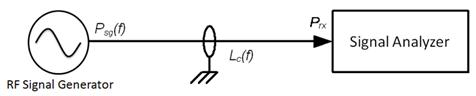Figure 4. Signal Analyzer Measuring Generator Error and Cable Loss

Figure 5 shows the absolute accuracy specification for an NI PXIe-5663. As shown, the typical measurement uncertainty is about + 0.65 dB.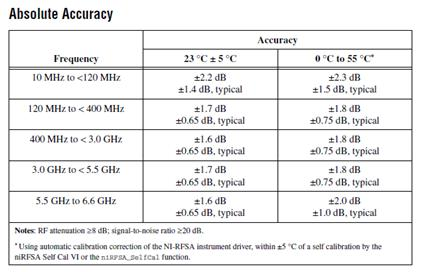Figure 5. NI PXIe-5663 Absolute Accuracy

With about 1.1 dB uncertainty from impedance mismatch and about 0.65 dB uncertainty in the analyzer's power measurement, the total uncertainty becomes the following: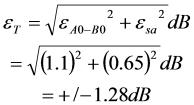Now consider the setup in Figure 4, which adds an attenuator with a frequency-dependent loss La(f) to the signal chain. The attenuator is often located near or directly attached to the UUT’s RF port to reduce the return loss. With a frequency-dependent combined cable loss of Lc(f), the RF signal generator power Psg is calculated as the following: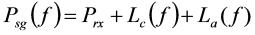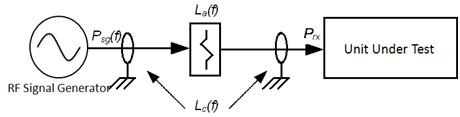Figure 6. Attenuator Added to Signal Chain

The below discussion of mismatch errors in Figure 7 makes the following assumptions:

• A good quality SMA attenuator is used with a return loss of < -30 dB.
• Again, the mismatch error caused by impedance mismatch between the RF signal generator and the power meter is ignored since power sensors normally have return loss < -25 dB. The overall contribution is very small compared to the mismatch error between the RF generator and the UUT.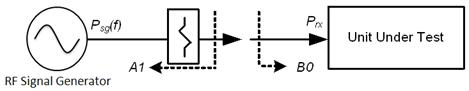Figure 7. Reference Planes With Attenuator

The attenuator improves the VSWR seen looking into A1 as much as the VSWR of the attenuator itself. Using 2-port analysis for the generator and attenuator circuit and applying Mason’s gain rule: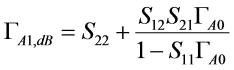And making the following assumptions,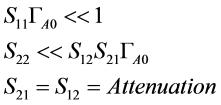The equation basically reduces the reflected waveform by the attenuation level two times: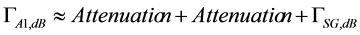Using an attenuator of 6 dB (VSWRatt = 1.05), the VSWRSG = 1.92 becomes VSWReq = 1.23. Figure 8 shows how different values of attenuators provide a lower mismatch uncertainty.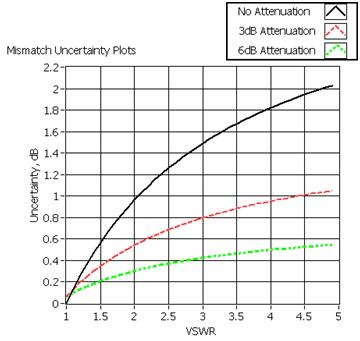Figure 8. How Mismatch Uncertainty Varies as the VSWR of the Source Device Increases (using NI PXIe-5673 signal generator)

The mismatch uncertainties between reference planes A1 (VSWR = 1.23) and B0 (VSWR = 2.5) are now the following: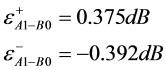Compare these mismatch uncertainties when using attenuation to the mismatch values when not using attenuation, calculated previously: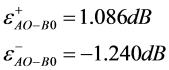Having shown the reduction of measurement uncertainty achieved by adding an attenuator to the signal chain, you can now focus on the measurement instrumentation. Power meters have better power measurement accuracy than signal or spectrum analyzers, but they typically do not have measurement ranges that extend below -40 dBm. Signal analyzers have the ability to measure much lower power signals (<-100 dBm), but they usually suffer from degraded accuracy at these low levels.

The implications of these facts are that if you need an RF measurement system to generate and measure both low- and high-power signals and responses, you may find yourself in a quandary. For example, assume the UUT has power-level test points of -30 dBm and -100 dBm. You can calibrate out the amplitude uncertainty of the generator (see Table 1) and the cable loss using a power meter attached to the generator output via the signal cable, with a test point of -40 dBm. This effectively transfers the accuracy of the power meter to the signal generator, assuming the generator configuration stays the same (for example, same power level, same frequency, no attenuator switches, and so on). You can compensate for the accuracy and cable loss error in the signal generator output, and you can place a 60 dBm attenuator at the UUT input, which provides a calibrated signal stimulus of -100 dBm at the UUT input.

However, to generate -30 dBm at the UUT input requires the signal generator to output roughly +30 dBm, a level not typically achievable without external amplification. This adds more complexity to the system and creates more system uncertainty. The limited ability of power meters to measure low-power signals creates dynamic range challenges for systems that need accurate power generation and measurement at low- and high-power levels, assuming a large fixed attenuator is used inline.

Before examining an advanced calibration procedure that you can use to transfer a power meter’s accuracy to both a signal generator and signal analyzer across a wide dynamic range using small attenuation values, explore an analysis of power meter measurement uncertainty as a foundation for future assertions.

## Power Meter Measurement Uncertainty

Critical to any calibration process involving a power meter is the understanding of the total measurement uncertainty of the power meter reading. This section discusses the variables of power meter measurement uncertainty, highlighting the most important. For example, an NI USB-5680 power meter is examined, though the concepts discussed extend to other power meters as well. For a complete analysis of USB-5680 uncertainty, as well as a link to a software tool for calculating USB-5680 measurement uncertainty refer to .

The first step in calculating the total USB-5680 measurement uncertainty is to calculate the uncertainties of the individual sources of measurement error. Figure 9 shows the various sources of measurement error for the USB-5680 at a frequency of 1 GHz at two different power levels of 0 dBm and -35 dBm. The three largest sources of error are impedance mismatch, linearity, and calibration factor, with mismatch typically the largest overall contributor. At lower levels within the power meter’s range, noise and zero errors become a larger relative contributor to overall measurement uncertainty. In addition, power meters themselves are typically calibrated at 0 dBm, so 0 dBm is a typical level used during an RF system calibration.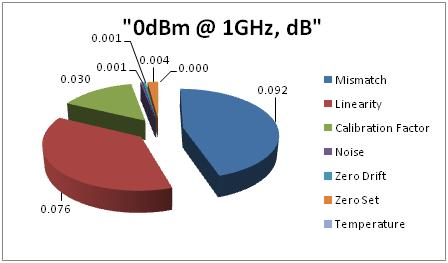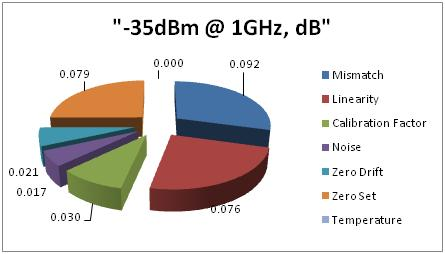Figure 9. USB-5680 Measurement Uncertainty Contributors at 1 GHz, 0 and -35 dBm

The important concept here is to normalize all uncertainties into their standard deviations. To do this, you need to know which type of probability distribution function each uncertainty has. Table 1 summarizes each uncertainty, the type of probability distribution the uncertainty follows, and the divisor that you can use to convert the uncertainty to one variance.

Table 1. USB-5680 Measurement Uncertainty Distributions and Associated Divisors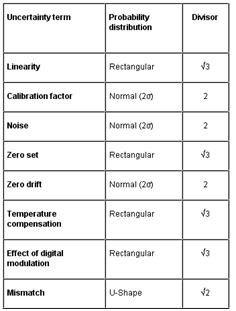The combined standard uncertainty is then calculated by combining the individual uncertainties as follows: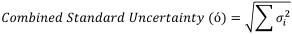Using  and the NI PXIe-5673 VSWR of 1.9 at 0 dBm and 1 GHz, you find that the total uncertainty is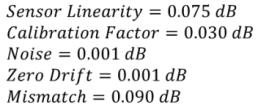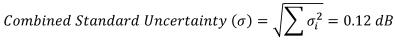The combined standard uncertainty calculated above represents + one standard deviation of the measurement uncertainty. To complete the uncertainty analysis, the expanded uncertainty is then calculated: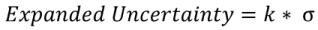The value k defines the desired confidence interval of the measurement. A normal distribution with a value k = 1 defines a confidence value of 68 percent, which is the confidence interval for the initial combined standard uncertainty calculation. A value k = 2 defines a 95 percent confidence interval within the normal distribution. Using a value of k = 2 provides an expanded measurement uncertainty value within which the power meter’s reading falls 95 percent of the time. See Figure 10 for a graphical representation of a normal distribution’s confidence intervals.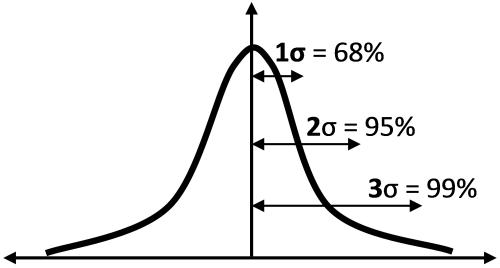Figure 10. Normal Distribution Confidence Intervals

Now that you understand the sources of uncertainty and know what the absolute accuracy is in the system, you can examine the calibration procedure in detail to characterize the full setup.

## Calibration Procedure Details

Figure 11 shows the test setup for the following advanced calibration procedure. Attenuators have been added to the input and output ports of the DUT to reduce error contributions due to impedance mismatches, as discussed previously. Also, the following discussion uses the NI PXIe-5673 RF vector signal generator, NI PXIe-5663 RF vector signal analyzer, and NI USB-5680 RF power meter as the instrumentation in the setup. You can make the following assumptions:

• Good quality SMA attenuators are used with return losses of < -30 dB.
• Again, the mismatch error caused by impedance mismatch between the RF signal generator and the power meter is ignored since power sensors normally have return loss < -25 dB. The overall contribution is very small compared to the mismatch error between the RF generator and the UUT.
• Return loss of cables and adapters is insignificant (in other words, only the VSWRs/impedances of the RF signal generator and UUT are considered).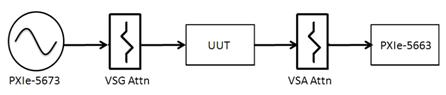Figure 11. Normal Distribution Confidence Intervals

This calibration procedure consists of three main steps:

1. Transfer power meter reference to signal generator at one power level.
2. Transfer signal generator reference to the signal analyzer at various power levels leveraging the linearity of the NI PXIe-5673 digital-to-analog converter (DAC).
3. Transfer signal analyzer references at various power levels back to the signal generator at various power levels.

The discussion of these steps includes screenshots of an NI TestStand sequence based on LabVIEW using the NI PXIe-5673, PXIe-5663, and USB-5680, as well as resultant data from this sequence.

#### Step 1—Transfer Power Meter Reference to Signal Generator

Figure 12 shows the setup for the first step of the calibration process. The purpose of this step is to essentially transfer the power meter accuracy to the signal generator over a range of frequencies and at a single power level. In place of the UUT, an SMA female-SMA female barrel adapter is attached to one end of the signal generator’s signal cable after the attenuator, which would normally be attached directly to the UUT. The power meter is connected to the other end of the SMA adapter. Assume that you are working with an ideal adapter with no impedance mismatch or insertion loss.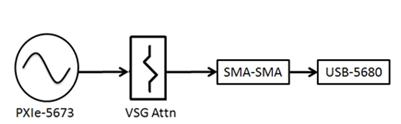Figure 12. Step 1 Setup

A power level of 0 dBm is used for this step since the USB-5680 is itself calibrated at 0 dBm. This is essentially the same process described at the beginning of this discussion, except the spectrum/signal analyzer has been replaced with a USB-5680 power meter.

This setup is familiar to many RF instrumentation users who use power meters to calibrate their signal generators. The difference is many of these users do not need to generate a wide range of power levels and can expect to operate their generators in power ranges that are also within the range of the power meters, in which case the power meters can be used as the measurement devices at all times. These users can simply perform this calibration procedure at each desired frequency and power test point in their test plans. The procedure described here transfers the power meter accuracy to the signal generator at a single power level. Ensuing steps describe the procedure for transferring this accuracy back to the signal analyzer at differing power levels. Again, the motivation for this procedure is to develop a method for obtaining power accuracy on par with a power meter across the wide range of the signal generator and signal analyzer.

Figure 13 shows a screen shot of the automated NI TestStand calibration sequence instructing the user to attach the USB-5680 to the other end of the SMA-SMA adapter.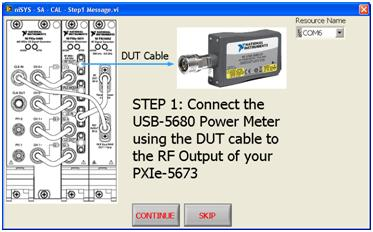Figure 13. NI TestStand Calibration Sequence Dialog—Step 1

Figure 14 shows data acquired from Step 1 over a frequency range of 1.8 GHz to 2.1 GHz.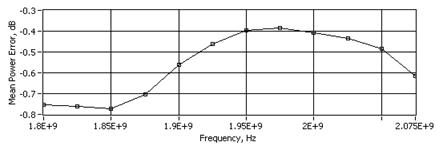Figure 14. Step 1 Signal Generator Calibration Data

#### Step 2—Transfer Reference From Signal Generator to Signal Analyzer

The next step in the procedure is to transfer the reference that has been effectively mapped from the power meter to the signal generator back to the signal analyzer. This is the unique portion of this procedure because it uses the linearity performance of the baseband arbitrary waveform generator’s (AWG’s) DAC to reliably transfer the measured reference to the signal analyzer.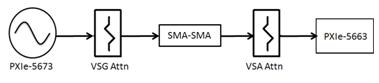Figure 15. Step 2 Setup

The NI PXIe-5450 dual-channel AWG is the baseband generator that composes one part of the overall NI PXIe-5673 vector signal generator (other components are the NI PXIe-5611 I/Q vector modulator and NI PXI-5652 RF signal generator, which acts as the LO source).

Step 1 provided a reference power measurement of the NI PXIe-5673 at the specific power level of 0 dBm. The measured power levels and associated offsets at each frequency capture the signal generator uncertainty at a particular frequency and output level. An intuitive approach in this step is simply to program the NI PXIe-5673 to sweep across the same frequency range but also across a set of desired power-level test points. However, programming the NI PXIe-5673 to output different power levels and simply reusing the same compensation values at these different power levels ignores the additional uncertainty introduced by nonlinearities of the signal generator output and the uncertainty from potentially different attenuator states.

Because the DAC linearity of the NI PXIe-5450 is better than the linearity of the NI PXIe-5673 RF output stage, you can use this with the NI PXIe-5673 module's "attenuator hold" feature to perform a very linear output power sweep with the NI PXIe-5673 by manipulating the output power digitally at the baseband stage rather than the RF stage via attenuators and RF amplifiers. The NI PXIe-5673 keeps all attenuators fixed in the same state at which the power meter reference measurement was obtained in Step 1. It then sweeps the power output downward simply by manipulating the NI PXIe-5450 DAC. At each of these steps, the signal analyzer is programmed with a new reference-level setting, so that the reference measurement obtained captures the current state of NI PXIe-5663 attenuator settings as well as the effects of the analyzer attenuator and analyzer signal cable path loss.

The result of this step effectively transfers the power meter reference obtained by the signal generator to the signal analyzer at multiple analyzer reference-level/attenuation settings. At the conclusion of this step, you have a signal analyzer calibrated at various signal levels and a signal generator calibrated at a single power level.

Figure 16 shows a screenshot of the automated NI TestStand calibration sequence instructing the user to attach the NI PXIe-5663 to the other end of the SMA-SMA adapter via the analyzer signal cable and attenuator.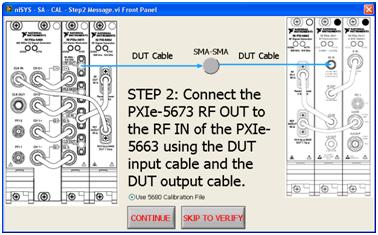Figure 16. NI TestStand Calibration Sequence Dialog—Step 2

#### Step 3—Transfer Reference From Signal Analyzer to Signal Generator

The final step in the procedure leaves the setup intact from Step 2 (see Figure 12). Step 3 involves calibrating the signal generator across multiple power levels using the signal analyzer you calibrated at various reference levels in Step 2.

The reason for this step is that you have calibrated the signal generator at a single output setting in Step 1, and, in Step 2, you calibrated the analyzer by varying the signal generator output digitally via the DAC. However, you have yet to calibrate the signal generator when the signal generator is programmed to output different power levels and the RF attenuators are allowed to change state.

Figure 17 shows some resultant data of the calibrated system when the generator and signal analyzer cables are connected directly via an SMA-SMA adapter, as shown in Figure 12.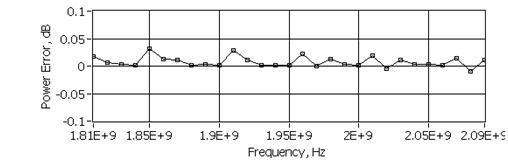Figure 17. Residual Error After System Calibration Across Different Frequencies at -10 dBm

#### Stability Over Temperature

An important consideration in all calibration situations is temperature drift. As temperature changes, it physically affects the entire system by changing cable and PWB trace lengths, increasing or decreasing thermal noise contributions, and making other changes as well. Ideally, the system operates in an environment whose temperature is exactly the same as the temperature when the system was calibrated. This section looks at the potential uncertainty added to your example test system using the NI 6.6 GHz RF platform and the USB-5680 power meter.

• The NI PXIe-5673 has a thermal drift of <0.2 dB/10 ˚C.
• The NI USB-5680 power meter has a thermal drift of <0.06 dB/50 ˚C.
• The NI PXIe-5663 has a thermal drift of <0.2 dB/10 ˚C.

Due to the small nature of these values, you can safely ignore temperature drift (depending on the application), provided the operating environment stays reasonably close (+2 degrees) to the calibration temperature.

Another consideration with respect to temperature is settling time. Every DUT and instrument has thermal settling time for the device. Figure 18 shows how the measured power changes over time. The calibration procedure you have discussed performs multiple measurements until the difference between two consecutive readings is below a threshold (set to 0.05 dB by default).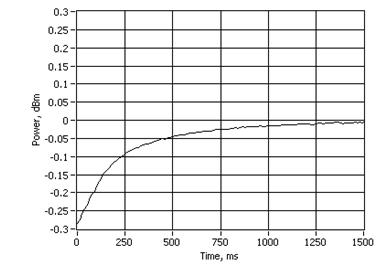Figure 18. Thermal Settling: If calibration is performed, the DUT should be settled.

#### Repeatability

The proposed method improves accuracy since the test setup has been characterized. For the frequencies and power levels at which it was characterized, the test system has the following experimental results.

Before characterizing the system, if you measure overall defined power levels and frequencies, the total error looks like Figure 19.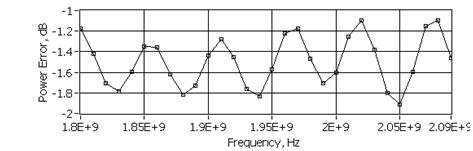Figure 19. Precalibrated Data Based on the Simple Approach: Effects due to cable loss, mismatch, and system accuracy are combined in the graph.

If you look at the histogram of Figure 19, you see (Figure 20) that the mean is about -1.5 dB (average cable loss). The standard deviation is 0.25 dB and the range is about 1.23 dB.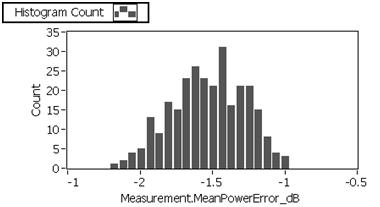Figure 20. Histogram of Precalibrated Data

Calculating the distribution of Figure 17 shows the following histogram (Figure 21).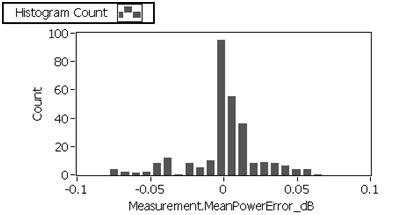Figure 21. Distribution of the Post-Calibration (Verification) Errors

This histogram has a mean at 0.008 dB, a standard deviation of 0.01 dB, and a range of 0.145 dB.

The presented data is the measured (composite) error of all the other variables that have different distributions. You can use this model to track what error is affecting the uncertainty the most and identify problems with the different setups.

## Conclusions

This paper has covered a simple yet complete method to perform a system calibration on NI RF instruments with the goal to improve system accuracy. To provide absolute measurements, the system was calibrated in base of a power meter. Total uncertainty values were presented and the experimental measurements show the typical absolute accuracy improvement.